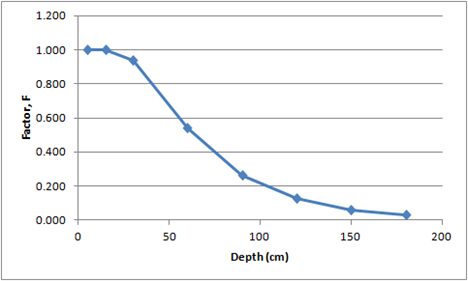# agmip.github.io

AgMIP open source software development and use.

View the Project on GitHub

# ROOT_DIST - Root distribution function

Distributes roots (or other soil parameters) using an exponential decay curve from a maximum value in the top soil to a minimum value at depth.

### Inputs:

• M = Maximum value in the top PP cm of soil (units depend on variable)
• PP = depth of top soil, or pivot point of curve (cm)
• RD = maximum rooting depth (cm), or depth at which the value is 2% of the maximum value

### Implicit Inputs (i.e., available in the data):

• SLLB = array of depths to bottom of soil layer (cm)

### Outputs:

• F = array of soil factors which decline exponentially between PP and RD (units depend on variable, same units as M)

### Procedure:

``````/* exponential decay rate */
k = LN(0.02)/(RD - PP)

/* loop thru soil layers */

/* midpoints of soil layers */
mid(1) = SLLB(1) / 2
mid(j) = [SLLB(j) + SLLB(j-1)] / 2

If mid(j) <= PP
then F(j) = M
else F(j) = M * EXP{k *(mid(j) - PP)}
``````

### Examples

#### Example 1 - Root growth factor:

``````FILL, SLRGF, ROOT_DIST(), 1.0, 20, 180
``````

For M = 1.0, PP = 20 cm, RD = 180 cm, the rate constant, k is calculated to be -0.02445 and the array of factors, F, are computed as:

sllb mid F
5 2.5 1.000
15 10 1.000
30 22.5 0.941
60 45 0.543
90 75 0.261
120 105 0.125
150 135 0.060
180 165 0.029More Samples: The following DOME spreadsheet uses this function: Machakos Overlay DOME

Dome functions page

Home